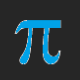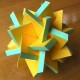# An Unsolved Problem Involving the Icosahedron and the Dodecahedron, and Their Circumscribed Spheres

This is apparently a problem, posed by Gregory Galperin, which went unsolved at the Bay Area Math Olympiad in 2005. I haven’t solved it yet, but I’m going to try, as I work on this blog-post. My 2010 source is a paper about Zome which may be read, as a .pdf, at bact.mathcircles.org/files/Summer2010/zomes-6-2010.pdf. The problem involves a dodecahedron and an icosahedron, each inscribed inside the same sphere of radius r, and asks which has the greater volume. At the time the authors wrote this paper, they knew of no solution, and I know of none now, but I do like a challenge.

My idea for solving this begins with Zome (info on Zome:  see http://www.zometool.com, as well as other sites you can find by googling “Zome”). In the Zome geometry system, using B1 struts for the edges of both a dodecahedron and an icosahedron, R1 struts are the radii of the circumscribed sphere for the icosahedron,  and Y2 struts are the radii for the circumscribed sphere of the dodecahedron. Since volume formula for polyhedra are generally given in term of edge-length, I need to find B1 in terms of R1 for the icosahedron, and find B1 in terms of Y2 for the dodecahedron.Icosahedron:  find B1, in terms of R1.

There exists a right triangle which can be built in Zome which has a hypotenuse equal to 2R1, and legs epqual to B1 and B2. B2 = φB1, so, by the Pythagorean Theorem, (2R1)^2 = (B1)^ + φ²(B1)², which simplifies to 4(R1)^2 = (1 + φ²)(B1)^2, which can then be solved for B1 as B1 = sqrt[4(R1)^2/(1 + φ²)]. B1 here is the icosahedron’s edge-length, while R1 is the radius of its circumscribed sphere.Dodecahedron:  find B1, in terms of Y2.

In the Zome system, Y2 = φY1, and Y1 = [sqrt(3)/2]B1. Rearrangement of the first of these equations yields Y1 = Y2/φ, and substitution then yields [sqrt(3)/2]B1 = Y2/φ, which then can be rearranged to yield B1 = 2Y2/[φsqrt(3)]. B1 here is the dodecahedron’s edge-length, while Y2 is the radius of its circumscribed sphere.

Next, find the volume of the icosahedron inscribed inside a sphere, in terms of that sphere’s radius.

According to http://mathworld.wolfram.com/Icosahedron.html, the volume of an icosahedron is given by V = (5/12)[3 + sqrt(5)]a³, where a is the edge length, or B1 in the first indented section, between the two images, above.  Then, by substitution, V = (5/12)[3 + sqrt(5)]{sqrt[4(R1)^2/(1 + φ²)]}³, which then becomes (with “r” being the radius of the circumscribed sphere) V = (5/12)[3 + sqrt(5)][2r/sqrt(1 + φ²)]³ = (40/12)[3 + sqrt(5)][1/sqrt(1 + φ²)]³r³ = (10/3)[3 + sqrt(5)][1/sqrt(1 + φ²)]³r³. Then, using the identity φ² = φ + 1, this can be further simplified to V = (10/3)[3 + sqrt(5)][1/sqrt(2 + φ)]³r³.

Next, find the volume of the dodecahedron inscribed inside the same sphere, in terms of that sphere’s radius, r.

According to https://en.wikipedia.org/wiki/Dodecahedron, the volume of an icosahedron is given by V = (1/4)[15 + 7sqrt(5)]a³, where a is the edge length, or B1 in the second indented section, below the second image, above.  Then, by substitution, V = (1/4)[15 + 7sqrt(5)]{2Y2/[φsqrt(3)]}³, which then becomes (with “r” being the radius of the circumscribed sphere) V = (8/4)[15 + 7sqrt(5)]{1/[φsqrt(3)]}³r³ = 2[15 + 7sqrt(5)]{1/[3sqrt(3)]}(1/φ³)r³ = (2/3)[15 + 7sqrt(5)][sqrt(3)/3](1/φ³)r³  = [2sqrt(3)/9][15 + 7sqrt(5)](1/φ³)r³.

So, with the “r” in each case being the same, the icosahedron is larger than the dodecahedron iff (10/3)[3 + sqrt(5)][1/sqrt(2 + φ)]³ > [2sqrt(3)/9][15 + 7sqrt(5)](1/φ³), which simplifies to (5)[3 + sqrt(5)][1/sqrt(2 + φ)]³ > [2sqrt(3)/3][15 + 7sqrt(5)](1/φ³), which simplifies further to {5/[sqrt(2 + φ)]³}[3 + sqrt(5)] > [2sqrt(3)/3φ³][15 + 7sqrt(5)], which is, as a decimal approximation, is (0.726542528)(5.2360679774998) > (3.464101615/12.708203932)(30.6524758), or 3.804226 > 8.355492, which is false, meaning that the dodecahedron is larger, not the icosahedron.

Now for the bad part:  I think I’m wrong, but I don’t know where the error lies. I’m also tired. If any of you see the mistake, please point it out in a comment, and I’ll try to fix this after I’ve rested.

Update:  if the websites http://rechneronline.de/pi/icosahedron.php and http://rechneronline.de/pi/dodecahedron.php work correctly, then the dodecahedron is larger. Evidence:This does not, however, mean that I did the problem correctly. I merely stumbled upon the correct answer. How do I know this? Simple:  the ratio I obtained was too far off. Therefore, I would still welcome help clearing up the mystery of where my error(s) is/are, in the calculations shown above.

## 7 thoughts on “An Unsolved Problem Involving the Icosahedron and the Dodecahedron, and Their Circumscribed Spheres”

1.chrismcmullen on said:

It looks like the dodecahedron is larger in the pictures, i.e. its faces seem to fit more smugly to the sphere. Intuitively, one might expect the solid with fewer sides to be smaller. But solids are complex: The number of edges joining at a vertex is quite important, too. The icosahedron has more vertices (these dual objects swap the 12 and 20 between vertices and faces). There are 5 triangles at a vertex, compared to three pentagons at a vertex. I think that’s why the dodecahedron appears to fit more snugly against the sphere. Not sure I have this right either (it’s late here, too). My first approach is to try and understand conceptually which should be larger. It’s an interesting puzzle.

Liked by 1 person

2.chrismcmullen on said:

Wait a minute. It looks like the solution to this problem is given in Eq. 21 of your Wolfram Mathworld reference, apparently solved by Apollonius. It gives the same ratio as your numerical example at the end of your article.

I’m thinking that the vertex solid angle should be proportional to the volume of a regular polyhedron inscribed in a sphere. I haven’t had a chance to work out the formula for it, though, to check, but it intrigues me.

Liked by 1 person

•RobertLovesPi on said:

Would that not make the volume of an inscribed tetrahedron much more than it actually is? Or am I missing something?

Like

•chrismcmullen on said:

The problem was surely in my explanation. 🙂

Let me use regular 2D polygons where there are fewer angles involved. The interior angles are 60 deg for triangles, 90 deg for squares, 108 deg for pentagons, 120 deg for hexagons, etc. As the number of sides increases, the interior angle increases, and the area of the inscribed polygon increases, right?

I expect for regular polyhedra that the “interior solid angle” (defined just like interior angle for polygons—place your eye at a vertex and look inward) will increase as the volume of the inscribed sphere increases (but the number of sides doesn’t go along with these). I would expect the “interior solid angle” to be a minimum for the tetrahedron and for the volume of the inscribed tetrahedron to a minimum, also. (I haven’t had a chance to work out any math thus far.)

Like

3.Robert Webb on said:

It’s easy enough to use Stella4D to show the volumes of each. The dodecahedron is larger. I would have expected the other way around, because the icosahedron has more and smaller faces, which you’d think would be pushed out towards the sphere more. But scaled this way, the icosahedron has longer edges, which moves its faces away from the sphere more.

My approach on paper would be to split them both into 120 right-tetrahedra, with these 4 corners: centre of polyhedron, centre of face, vertex, and centre of edge; and just calculate the volume of one of those for the two shapes. Well, that’s a start anyway.

Liked by 1 person

4.Anonymous on said:

Retroductive logic solves more efficiently

Like

5.Anonymous on said:

This question has ancient origins. In the Timaeus, Plato identified four of the five regular solids with the four elements (Fire, Air, Water, Earth), and the fifth with the Universe as a whole. The reason Plato chose the dodecahedron to represent the universe as a whole is that it most closely approximates the volume of a sphere, which was believed to be the shape of the universe at the time. (For the other four: fire = tetrahedron, air = octahedron, water = icosahedron, earth = cube.)

Liked by 1 person